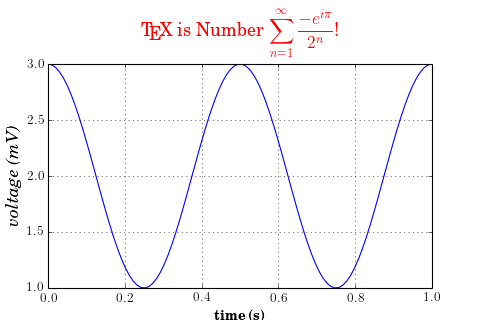# pylab_examples example code: tex_demo.py¶"""
You can use TeX to render all of your matplotlib text if the rc
parameter text.usetex is set.  This works currently on the agg and ps
backends, and requires that you have tex and the other dependencies
described at http://matplotlib.org/users/usetex.html
properly installed on your system.  The first time you run a script
you will see a lot of output from tex and associated tools.  The next
time, the run may be silent, as a lot of the information is cached in
~/.tex.cache

"""
import numpy as np
import matplotlib.pyplot as plt

plt.rc('text', usetex=True)
plt.rc('font', family='serif')
plt.figure(1, figsize=(6,4))
ax = plt.axes([0.1, 0.1, 0.8, 0.7])
t = np.linspace(0.0, 1.0, 100)
s = np.cos(4 * np.pi * t) + 2
plt.plot(t, s)

plt.xlabel(r'\textbf{time (s)}')
plt.ylabel(r'\textit{voltage (mV)}',fontsize=16)
plt.title(r"\TeX\ is Number $\displaystyle\sum_{n=1}^\infty" r"\frac{-e^{i\pi}}{2^n}$!", fontsize=16, color='r')
plt.grid(True)
plt.savefig('tex_demo')
plt.show()


Keywords: python, matplotlib, pylab, example, codex (see Search examples)